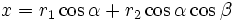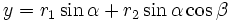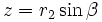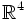# Difference between revisions of "Torus in 3-space"

Jump to: navigation, search

## Definition

The torus in$\R^3$ is constructed as follows:

• Take a base circle centered at a point which we shall call the origin. We shall call the radius of the base circle the average radius.
• Fix a length smaller than the average radius, called the tube radius.
• For each point on the torus, consider the circle centered at that point of radius equal to the tube radius, in the plane perpendicular to the plane of the base circle, and containing that center and the origin
• The union of all such circles is termed the torus

The torus can thus be thought of as the trace of a circle whose center is itself moving on a base circle, such that the plane of the circle always contains the origin of the base circle.

Some further terminology:

• The inner radius is the average radius minus the tube radius. It is the shortest possible distance between the origin and points on the torus. The set of points at this minimum distance forms a circle, called the inner rim or inner circle.
• The outer radius is the average radius plus the tube radius. It is the maximum distance between the origin and points on the torus. The set of points at this maixmum distance form a circle, called the outer rim or outer circle.
• Consider two planes parallel to the plane containing the base circle, with distance from it equal to the tube radius. Both these planes are tangent to the torus, meeting it at circles. These circles are termed the top circle and bottom circle (interchangeably). The radius of these circles is equal to the average radius.

## Equational descriptions

### Cartesian parametric equation

Let$r_1$ denote the average radius and$r_2$ denote the tube radius. Suppose the base circle is in the$xy$-plane and the origin of the torus is the origin. Then the parametric equations aer in terms of two angles,$\alpha$ and$\beta$, where:

•$x = r_1 \cos \alpha + r_2 \cos \alpha \cos \beta$
•$y = r_1 \sin \alpha + r_2 \sin \alpha \cos \beta$
•$z = r_2 \sin \beta$

Both$\alpha$ and$\beta$ are modulo$2\pi$.

## Abstract structure

Topologically, and even differentially, the torus is isomorphic to the direct product of circles, viz$S^1 \times S^1$. One coordinate describes the position of the center on the base circle, and the other coordinate describes the position of the point on that particular small circle. The Cartesian parametric equations given above make this explicit.

However, the metric structure on the torus is very different from that on$S^1 \times S^1$ with the direct product metric. The latter is actually the flat torus, which cannot be embedded in$\R^3$, but needs to be embedded in$\R^4$.

## Structure and symmetry

The isometry group of the torus is the semidirect product of the following two groups:

• The group$S^1$, which acts on the first coordinate by rotating the base circle.
• The group of order two which reflects the whole configuration about the$xy$-plane

In fact, this is precisely the group of isometries of$\R^3$ which fix the origin and the preserve the$z$-axis (though they may not preserve direction).